Home > CC3 > Chapter 9 > Lesson 9.1.3 > Problem9-35

9-35.
1. Simplify each expression. Homework Help ✎

1.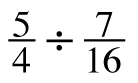2.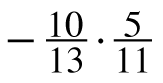3.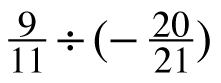4.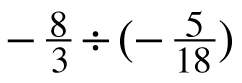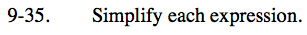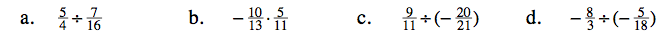When you divide by a fraction, you are also multiplying by its reciprocal.

$\frac{5}{4} \cdot \frac{16}{7}$

$\frac{20}{7}$

The answer will be negative.

See (a).

$\frac{9}{11} \cdot \left(-\frac{21}{20}\right)$

See the hints for part (a).

$\frac{48}{5}$